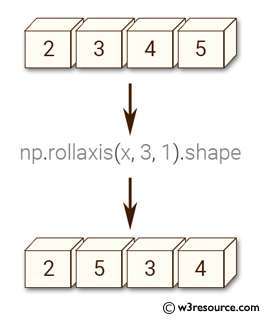﻿ NumPy: Move the specified axis backwards, until it lies in a given position - w3resource# NumPy: Move the specified axis backwards, until it lies in a given position

## NumPy: Array Object Exercise-53 with Solution

Write a NumPy program to move the specified axis backwards, until it lies in a given position.
Move the following 3rd array axes to first position.
(2,3,4,5)

Pictorial Presentation:Sample Solution:-

Python Code:

``````import numpy as np
x = np.ones((2,3,4,5))
print(np.rollaxis(x, 3, 1).shape)
```
```

Sample Output:

```(2, 5, 3, 4)
```

Python Code Editor:

Have another way to solve this solution? Contribute your code (and comments) through Disqus.

What is the difficulty level of this exercise?

Test your Python skills with w3resource's quiz

﻿

## Python: Tips of the Day

Set comprehension:

```>>> m = {x ** 2 for x in range(5)}
>>> m
{0, 1, 4, 9, 16}
```